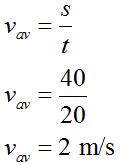# Define and calculate average speed and velocityWhat is the average speed of a car that travels a distance of 40.0 m in a time of 20.0 seconds?a.2.00 m/s b.0.500 m/s c.80.0 m/s d.0.125 m/s e.800 m/s

Question
2 views

Define and calculate average speed and velocity

What is the average speed of a car that travels a distance of 40.0 m in a time of 20.0 seconds?

 a. 2.00 m/s b. 0.500 m/s c. 80.0 m/s d. 0.125 m/s e. 800 m/s
check_circle

Step 1

1)

Average Speed

Average speed is defined as the ratio of total distance travelled to the total time to cover the distance.

Average Velocity

Average velocity is defined as the ratio of displacement to time taken in total distance. displacement does not depend on the way of path it is just difference of inital position to final position of an object. velocity is a vector quantity because the displacement is a vector quantity.

Step 2

2)

Given:

distance travelled by car (s) = 40.0 m.

time taken to cover the distance (t) = 20.0 s.

Step 3

Average speed will be,...

### Want to see the full answer?

See Solution

#### Want to see this answer and more?

Solutions are written by subject experts who are available 24/7. Questions are typically answered within 1 hour.*

See Solution
*Response times may vary by subject and question.
Tagged in

### Physics